﻿
﻿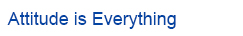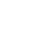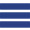How to set the high precision standard of Bewis tilt sensor Release date：2020-03-12   Browsing volume：299

Many customers are often at a loss when facing many parameters such as accuracy, cross-axis error, and temperature drift when selecting an inclination sensor, and little is known about the test standards for each parameter. How to start with accuracy and choose a suitable one What about the product? Taking Bewis sensor accuracy evaluation standards as an example, I will give you a related introduction.

There are many ways to measure, and the commonly used methods of measuring accuracy are standard deviation and root mean square error.

Standard deviation (1) is divided into sample standard deviation and population standard deviation. The formula for calculating the sample standard deviation is as follows: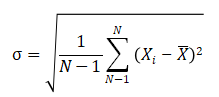Standard deviation reflects the degree of dispersion of a finite data set.

The root mean square error (RMSE), also known as standard error, calculates the degree of dispersion of the measured value in the predicted value. The formula is: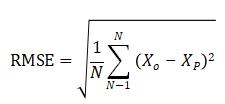The number of observations n collected in the actual measurement test is limited, and the true value can only be replaced by the most reliable (best) value.

Bewis sensing high-precision tilt sensor has two calculation methods for calculating accuracy. The first method is to collect a value every 2-3 ° in the relative accuracy range, and then subtract the current observation value to use the remaining data for accuracy. For calculation, the second method is to take a fixed value gradient calculation and collect 16 values for calculation at a fixed angle.

Now we use the second accuracy calculation method, taking Bewis sensor BWM826 as an example. The related parameters of BWM826 are as follows:Turn the high-precision three-axis turntable to the X-axis of 5 ° and the Y-axis of 0 °. The acquisition sensor is powered on for 3 minutes and the data is collected for 15 minutes for analysis. The data is shown in the figure: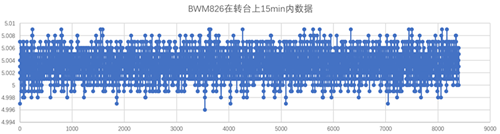A total of 8399 data were collected in 15 minutes, and the average was: 5.00334. The accuracy comparison chart obtained by using the sample standard deviation and root mean square error calculation method for different sets of data is as follows: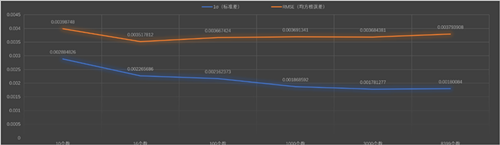In order to avoid accidents, 16 numbers were collected at different positions of the data and the root mean square error was calculated: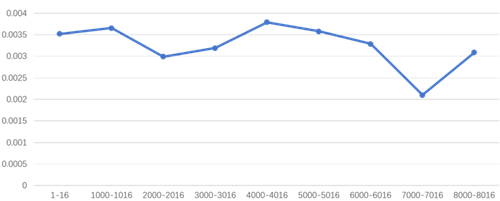From the two figures above, it can be found that the accuracy of the root mean square error and the standard deviation calculation can reflect the accuracy of the BWM826 sensor (0.005 °), but the accuracy calculated by the root mean square error is worse than the accuracy calculated by the standard deviation. Because the standard deviation meter calculates the data set to fluctuate above and below the mean (5.00334).

If you take 5.00334 as a reference, most of this set of data is within ± 0.005 °, that is, 4.9984-5.00834, but relatively speaking it can not accurately reflect the deviation from 5 °, and the root mean square error is reflected when 5 ° is reflected. There is a fluctuation, so Bewis sensor uses the root mean square error to measure the accuracy of the sensor.

Bewis sensing high-precision inclination sensors have strict accuracy standards. Through correct accuracy calculation methods, the performance of each product can be distinguished. Based on this, it provides the most suitable inertial attitude sensors for various industries, and also for the industry Application brings convenience.

TAG：
• STAY CONNECTED
• 24 Hours Service Online

WhatsApp/Tel：+86 15050 672 146

+86 17606 118 008

Commercial：sales@bwsensing.com

Customer Service：support@bwsensing.com

•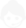•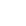+86 189 2129 2620
•sales@bwsensing.com
•